# The GUE hypothesis

Problem: Formulate a precise and believable statement of the GUE Hypothesis.''

Any reasonable form of the conjecture should predict that the correlation functions of the zeros and the distribution of the neighbor spacings of the zeros of any automorphic L-function should have the same statistics as the eigenvalues of some collection of matrices.

The question is: which statistics? what collection of matrices (which may be a function of how high up' the zeros are)? what is the rate of convergence, and how uniform is it in the various parameters?

Montgomery's original pair correlation conjecture was that if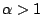then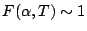as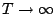, uniformly for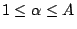. A weak version of the conjecture asserts that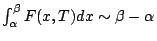, uniformly for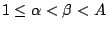. Such an `almost everywhere'' version is usually all that is needed for applications. Goldston and Montgomery [ MR 90h:11084] show that it is equivalent to a statement about the variation of the distribution of primes in short intervals, and Goldston, Gonek, and Montgomery (to appear in Crelle) show tht it is equivalent to a statement about the mean square of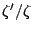near the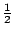-line.

It is possible that an acceptable formulation of the GUE conjecture can be made in terms of integrals of ratios of $\zeta$-functions.

Back to the main index for L-functions and Random Matrix Theory.